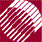ECE532 Biomedical Optics © 1998 Steven L. Jacques, Scott A. Prahl Oregon Graduate Institute

This header section of the program lists various details necessary for a C program to run. It's not so interesting and you can skip ahead to the next section unless you really want to read the details.

The "include" statements incorporate standard subroutine libraries available with any ANSI standard C compiler.

#include <math.h>
#include <stdio.h>

The "define" statements make global substitutions throughout the program before compiling the program. Hence the progam source code can use simpler names like PI, ALIVE, ONE_MINUS_COSZERO, for easier reading.

A subroutine called RandomGen() is used to generate random numbers between 0 and 1 inclusive. "InitRandomGen" is substituted by a call to RandomGen(0, 1, NULL) which initializes the seed for the random number generator subroutine. "RandomNum" is substituted by a call to RandomGen(1, 0, NULL) which calls for a random number. The function "RandomGen()" is declared, and is listed at the end of the program. In the main program, "InitRandomGen" initializes RandomGen(), and subsequent uses of "RandomNum" calls for a random number from RandomGen().

#define	PI          3.1415926
#define ALIVE       1   		/* if photon not yet terminated */
#define DEAD        0    		/* if photon is to be terminated */
#define THRESHOLD   0.01		/* used in roulette */
#define CHANCE      0.1  		/* used in roulette */
#define COS90D      1.0E-6
/* If cos(theta) <= COS90D, theta >= PI/2 - 1e-6 rad. */
#define ONE_MINUS_COSZERO 1.0E-12
/* If 1-cos(theta) <= ONE_MINUS_COSZERO, fabs(theta) <= 1e-6 rad. */
/* If 1+cos(theta) <= ONE_MINUS_COSZERO, fabs(PI-theta) <= 1e-6 rad. */
#define SIGN(x)           ((x)>=0 ? 1:-1)
#define InitRandomGen    (double) RandomGen(0, 1, NULL)
/* Initializes the seed for the random number generator. */
#define RandomNum        (double) RandomGen(1, 0, NULL)
/* Calls for a random number from the randum number generator. */

/* DECLARE FUNCTION */
double RandomGen(char Type, long Seed, long *Status);
/* Random number generator */

The main program is contained with a section called "main()". Following "main()", the function RandomGen() is listed.

main() {

/**** PROGRAM SITS HERE ****/

}

/* FUNCTION */

/**** RandomGen() function listed here ****/

to next page | Chapter 4 | Course | Home

©1998, Steven L. Jacques, Scott A. Prahl, Oregon Graduate Institute, Oregon Medical Laser Center